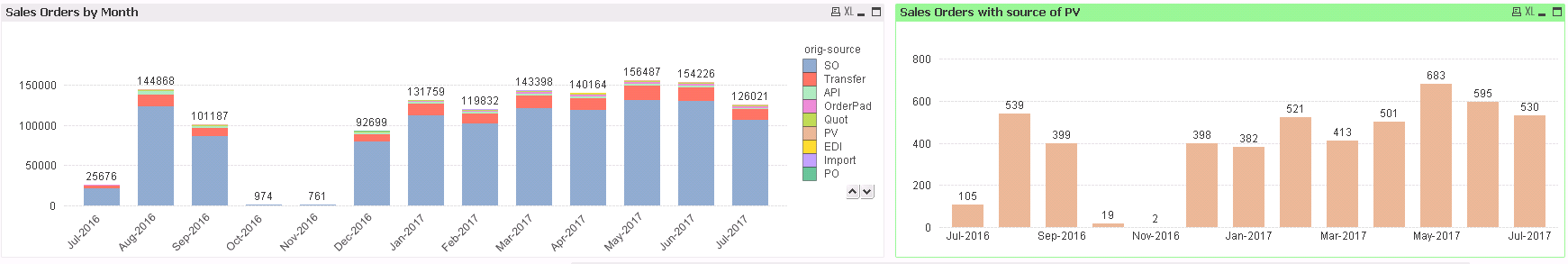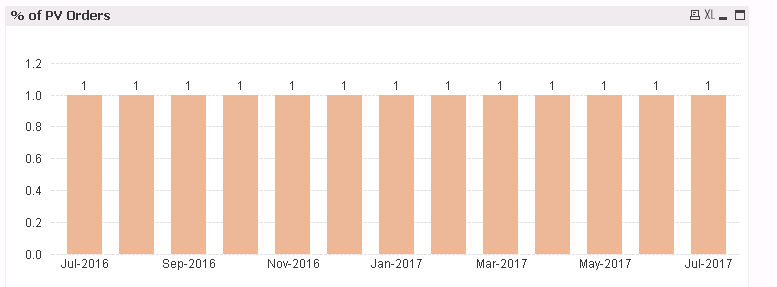# New to QlikView

Discussion board where members can get started with QlikView.

HighlightedNot applicable

## Can't divide two calculations in chart

This is extremely weird, or maybe I'm just doing something really simple, really wrong.

Here are two charts. One is total orders, one is total orders with a source of PV.Here are the two expressions.

I want a chart that shows % of PV Orders from Total orders.

Naturally, I use this.

/

But this is my chart...Why?

Tags (6)
1 Solution

Accepted Solutions
Contributor II

## Re: Can't divide two calculations in chart

Hi Aaron,

Try below expression -

/

6 Replies
Contributor II

## Re: Can't divide two calculations in chart

Hi Aaron,

Try below expression -

/

New Contributor III

## Re: Can't divide two calculations in chart

As Milind wrote, the set analysis in the denominator is useless, because you need to divide in the total count and not the selected count.MVP

## Re: Can't divide two calculations in chartNot applicable

## Re: Can't divide two calculations in chart

Thanks!Not applicable

## Re: Can't divide two calculations in chart

I didn't think of that, thanksNot applicable

Thanks!